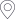﻿ 蔡好涛教授学术报告-烟台大学 数学与信息科学学院首页 > 科学研究 > 学术报告 > 正文

## 蔡好涛教授学术报告

The classical integer-order Jacobi spectral methods for solving second kind nonlinear Volterra integral equations with weakly singular kernels may cause a low-order accuracy in numerically approximating the exact solution. To overcome the shortcomings, we in this paper present a fractional spectral collocation method for solving weakly singular nonlinear Volterra integral equations. Based on the behavior of the original solution near the initial point of integration, we construct the fractional interpolation basis in the collocation method, and then develop an easily implementing technique to approximate the entry with one-fold integral in the resulting nonlinear system produced by the fractional spectral method. Consequently, we establish that both the semi-discrete and the fully discrete nonlinear systems have a unique solution for sufficiently large \$n\$, respectively, where \$n+1\$ denotes the dimension of the approximate space. We also ensure that two approximate solutions produced by both the semi-discrete and the fully discrete method arrive at the quasi-optimal convergence order in the infinite norm. At last, numerical examples are given to confirm the theoretical results.